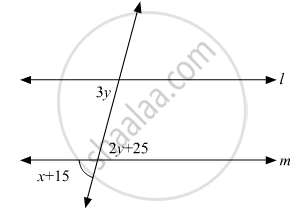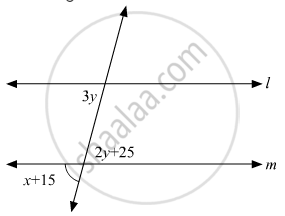# In the Given Figure, If L || M, What is the Value of X? - Mathematics

MCQ

In the given figure, if l || m, what is the value of x?• 60°

• 50°

• 45°

• 30°

#### Solution

Given figure is as follows:Since (x + 15°)and (2y + 25°)are vertically opposite angles, therefore,

(x + 15) = (2y + 25)

 x -2y = 25° - 15°

 x - 2y = 10°               (1)

Also, 3y and (2y + 25°)are alternate interior opposite angles, therefore,

3y = (2y + 25°)

3y - 2y = 25°

 y = 25°

Substituting in equation (i), we get:

x - 2(25°) = 10°

x - 50° = 10°

x = 50° + 10°

x = 60°

Concept: Introduction to Lines and Angles
Is there an error in this question or solution?
Chapter 10: Lines and Angles - Exercise 10.6 [Page 55]

#### APPEARS IN

RD Sharma Mathematics for Class 9
Chapter 10 Lines and Angles
Exercise 10.6 | Q 18 | Page 55
Share